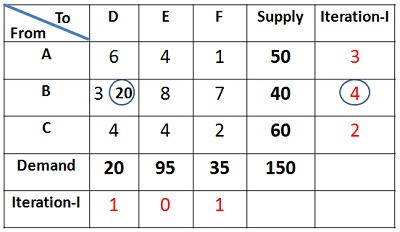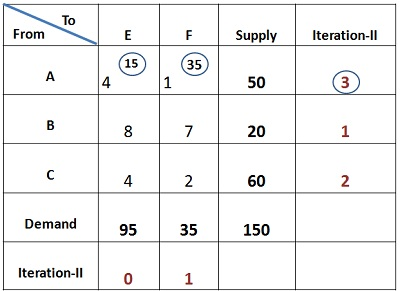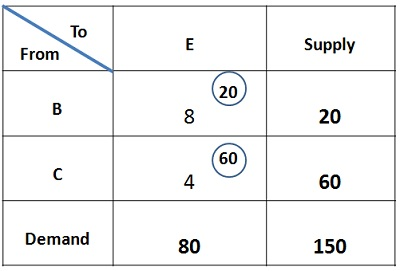# Vogel’s Approximation Method

The Vogel’s Approximation Method or VAM is an iterative procedure calculated to find out the initial feasible solution of the transportation problem. Like Least cost Method, here also the shipping cost is taken into consideration, but in a relative sense.

The following is the flow chart showing the steps involved in solving the transportation problem using the Vogel’s Approximation Method:The concept of Vogel’s Approximation Method can be well understood through an illustration given below:

1. First of all the difference between two least cost cells are calculated for each row and column, which can be seen in the iteration given for each row and column. Then the largest difference is selected, which is 4 in this case. So, allocate 20 units to cell BD, since the minimum cost is to be chosen for the allocation. Now, only 20 units are left with the source B.2. Column D is deleted, again the difference between the least cost cells is calculated for each row and column, as seen in the iteration below. The largest difference value comes to be 3, so allocate 35 units to cell AF and 15 units to the cell AE. With this, the Supply and demand of source A and origin F gets saturated, so delete both the row A and Column F.3. Now, single column E is left, since no difference can be found out, so allocate 60 units to the cell CE and 20 units to cell BE, as only 20 units are left with source B. Hence the demand and supply are completely met.Now the total cost can be computed, by multiplying the units assigned to each cell with the cost concerned. Therefore,

Total Cost = 20*3 + 35*1 + 15*4 + 60*4 + 20*8 = Rs 555

Note: Vogel’s Approximation Method is also called as Penalty Method because the difference costs chosen are nothing but the penalties of not choosing the least cost routes.

## 4 thoughts on “Vogel’s Approximation Method”

error: Content is protected !!